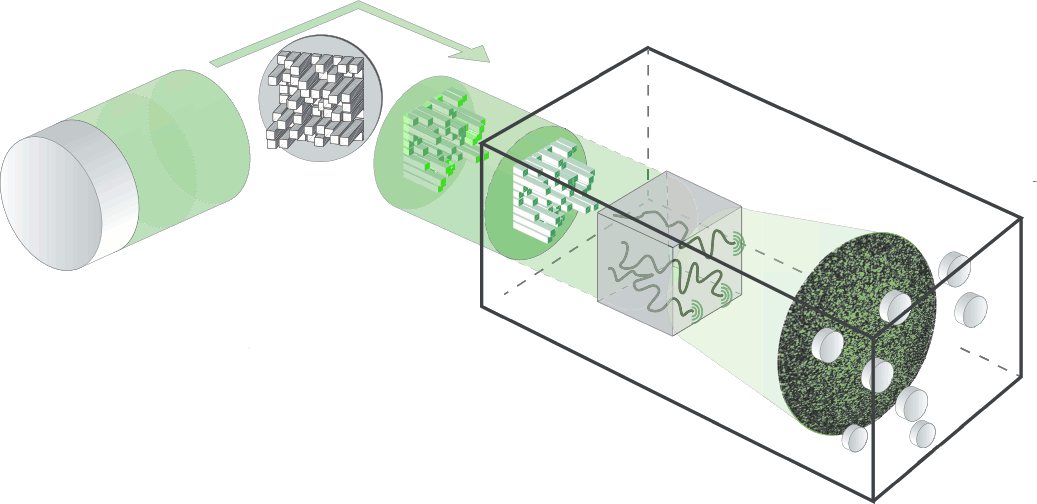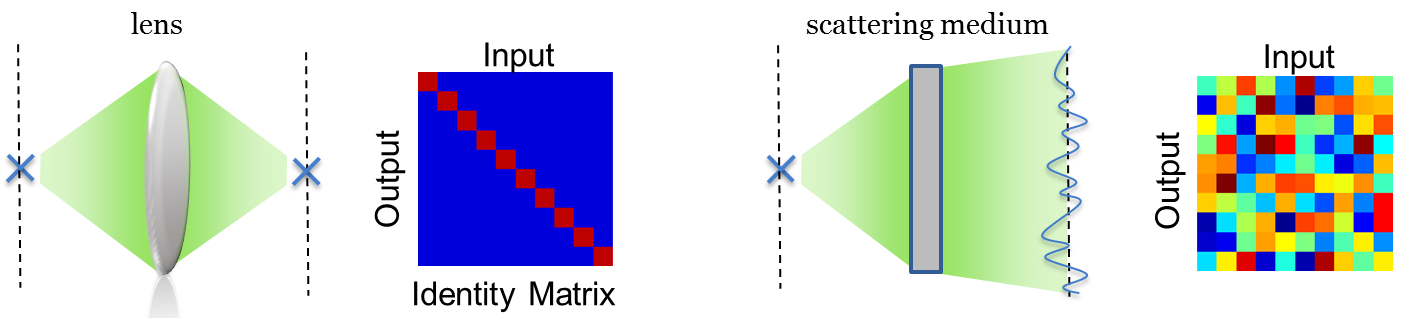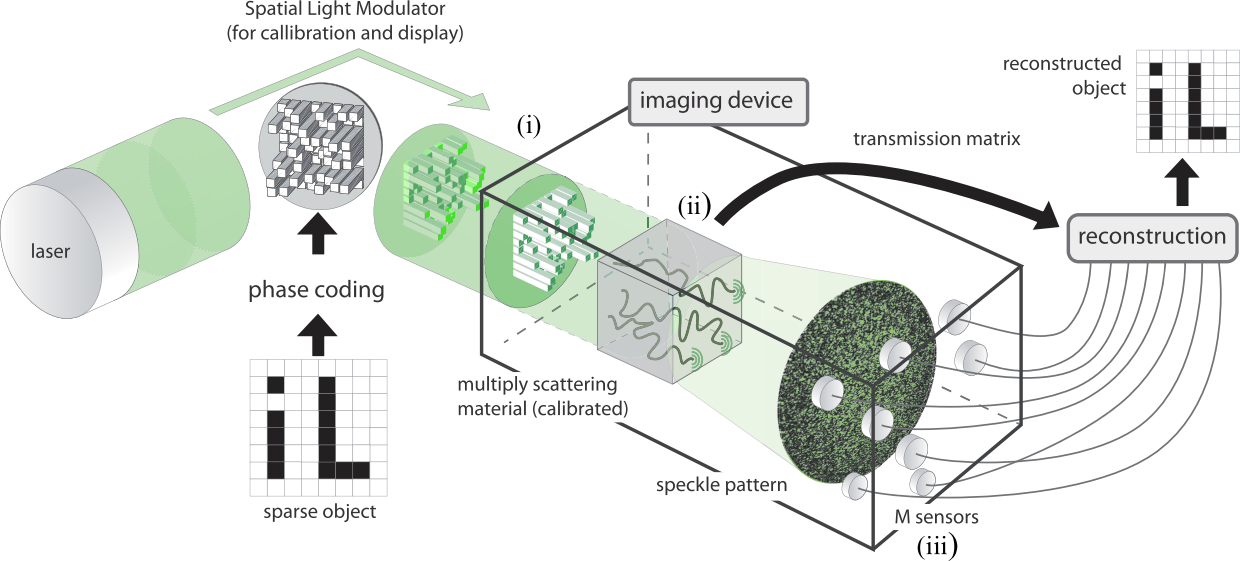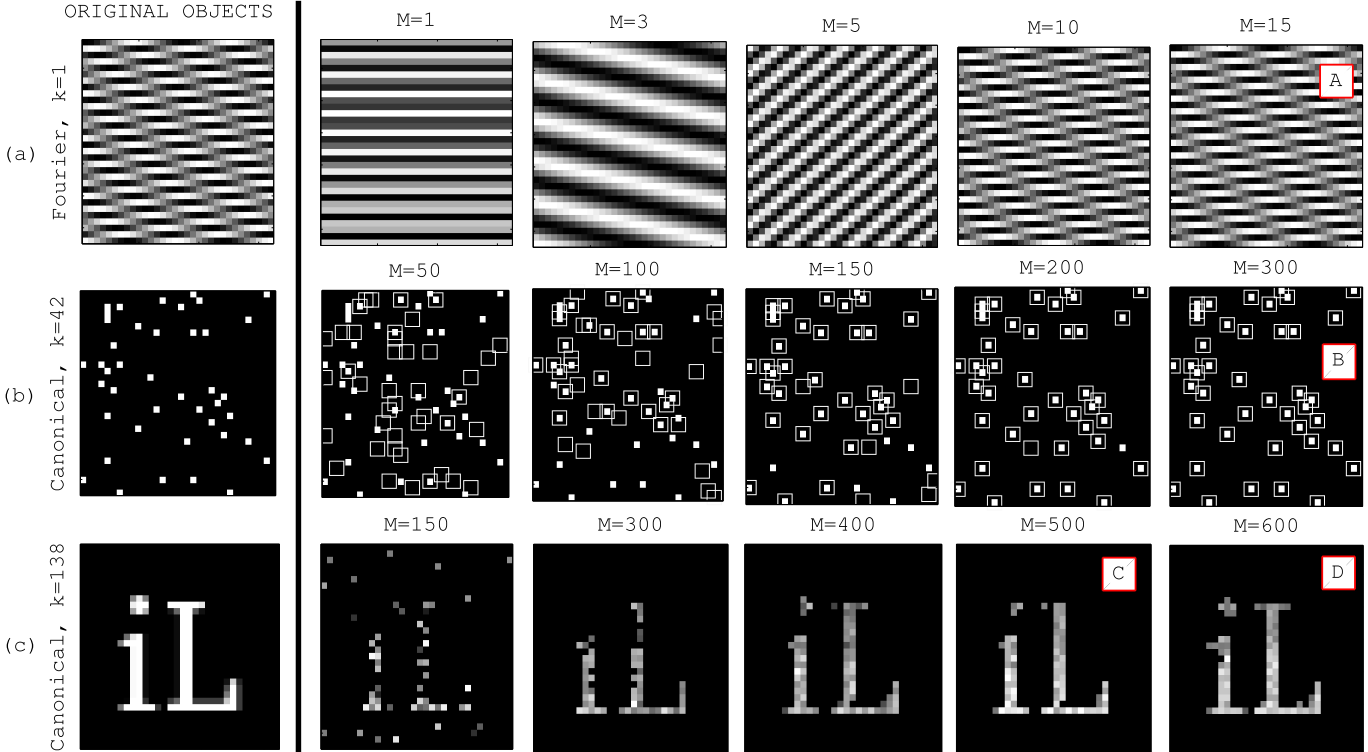## Imaging with nature: Using a scattering medium as a universal scrambler for imaging by compressed sensing[A. Liutkus et al., Sci. Rep. 5, (2014)]

The idea of compressive sensing is to acquire an image with fewer measurements than dictated by the Shannon-Nyquist theorem. In other words, an image divided into "pixels" can usually be reconstructed using fewer measurements than the total number of pixels. To do so, one needs a way to mix the information, so that any measurement contains at least a bit of information on any input element. Previous implementations of compressive sensing consisted of artificially designing hardware and a sampling procedure to generate randomness. In the present paper, the authors show that one can use a random scattering medium as a universal image scrambler. The light reflected from an image propagates through a layer of white paint and the field is measured on different receptors on the other side of the sample. By previously measuring the transmission matrix, the authors show that sparse images can be successfully reconstructed using compressed sensing techniques taking advantage of the randomness generated by multiple scattering.

In a standard optical system, the intensity measured by one pixel of a camera probes the intensity on a small finite area in the object plane. The transmission matrix between the object plane and the image plane is an identity matrix (see Fig. 1(a)). Reducing the number of pixels or receptors in the image plane corresponds to a loss of information because the information carried by a missing pixel is not present anywhere else. A scattering medium has the property to randomly mix the light, a local excitation at the input of the medium gives rise to a broad random speckle pattern on the output as represented in Fig. 1(b). The transmission matrix seems random, it is this very property that is sought for compressed sensing; any output element statistically carries a bit of information coming from all the input excitation positions.Figure 1. Standard imaging (a.) versus scattering medium (b.) and their associated transmission matrices.

To calibrate the system, the authors measured the transmission matrix of the scattering system - a 300 µm thick layer of zinc oxide. This matrix links the optical field on the pixels of the spatial light modulator (SLM) used to modulate input light to the field on the pixels of the CCD camera used to probe output light. For a set of input illuminations, the complex output field is measured to build the transmission matrix. The technique is slightly different from the one initially presented in  to estimate more precisely the matrix in the presence of noise. A schematic of the experiment is presented in Fig. 2.Figure 2. Schematic of the experiment. Image from [A. Liutkus et al., Sci. Rep. 5, (2014)]

Ideally, the transmission matrix that links the field on the input plane to the receptors has to be as random as possible to maximize the efficiency of compressed sensing techniques. A random matrix with identically and independently distributed random elements is one of the best candidates . It has been shown recently that the transmission matrix of a scattering sample where only a random partition of the output modes are measured satisfies these conditions  (see From the bimodal distribution to...). In other words, the matrix that links the elements of the modulator to the receptors randomly positioned behind the medium is mathematically ideal for an image reconstruction using compressed sensing.

The reconstruction of sparse images in different basis is tested with different numbers M of receptors used. The results are shown in Fig. 3. In each case, the reconstruction needs fewer receptors than the 1024 pixels of the image. The authors show in the paper that the efficiency of the reconstruction is close to the theoretical predictions for an optimal random matrix. Once again, the advantage of this technique is that it does not need any effort to generate the randomness - that is here provided by the random nature of the scattering sample.Figure 3. Example of images reconstructed by compressed sensing. Image from [A. Liutkus et al., Sci. Rep. 5, (2014)]

 S.M. Popoff et al., Phys. Rev. Lett., 104, (2010)

 D. Donoho et al., Proc. IEEE., 98, (2010)

 A. Goetschy et al., Phys. Rev. Lett., 111, (2013)

Created by sebastien.popoff on 09/09/2013